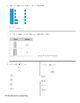# First Grade Go Math Chapter 8 Review HomeworkSubject
Resource Type
File Type

PDF

(1 MB|13 pages)
Product Rating
Standards
Also included in:
1. This is a bundle that includes all 12 first grade Go Math! chapter review assignments. Each review can be used as a homework assignment, an in class review, or another form of the third grade chapter test. Each chapter review includes the same amount of questions as the review/test from the book, as
\$36.00
\$25.00
Save \$11.00
2. This is a GROWING bundle for ALL Kindergarten-5th Grade Go Math! chapter resources I have created thus far. These math reviews are correlated to the Go Math! series. This GROWING bundle is geared towards those that teach math to multiple grade levels, switch grade levels frequently, or need to diffe
\$207.00
\$143.00
Save \$64.00
• Product Description
• StandardsNEW

All 12 of my first grade Go Math Chapter Review Homework products (including this file) are included in my First Grade Go Math Chapters 1-12 Review Homework BUNDLE for a discounted price.

These questions are based on what was learned in the Chapter 8 Go Math! first grade curriculum. This can be used as a homework assignment, an in class review, or another form of the first grade Chapter 8 Go Math chapter test. It includes 12 questions just like the review/test from the book, as well as, the chapter test. Includes an answer key.

Add and subtract within 20, demonstrating fluency for addition and subtraction within 10. Use strategies such as counting on; making ten (e.g., 8 + 6 = 8 + 2 + 4 = 10 + 4 = 14); decomposing a number leading to a ten (e.g., 13 - 4 = 13 - 3 - 1 = 10 - 1 = 9); using the relationship between addition and subtraction (e.g., knowing that 8 + 4 = 12, one knows 12 - 8 = 4); and creating equivalent but easier or known sums (e.g., adding 6 + 7 by creating the known equivalent 6 + 6 + 1 = 12 + 1 = 13).
Subtract multiples of 10 in the range 10-90 from multiples of 10 in the range 10-90 (positive or zero differences), using concrete models or drawings and strategies based on place value, properties of operations, and/or the relationship between addition and subtraction; relate the strategy to a written method and explain the reasoning used.
Add within 100, including adding a two-digit number and a one-digit number, and adding a two-digit number and a multiple of 10, using concrete models or drawings and strategies based on place value, properties of operations, and/or the relationship between addition and subtraction; relate the strategy to a written method and explain the reasoning used. Understand that in adding two-digit numbers, one adds tens and tens, ones and ones; and sometimes it is necessary to compose a ten.
Total Pages
13 pages
Included
Teaching Duration
N/A
Report this Resource to TpT
Reported resources will be reviewed by our team. Report this resource to let us know if this resource violates TpT’s content guidelines.